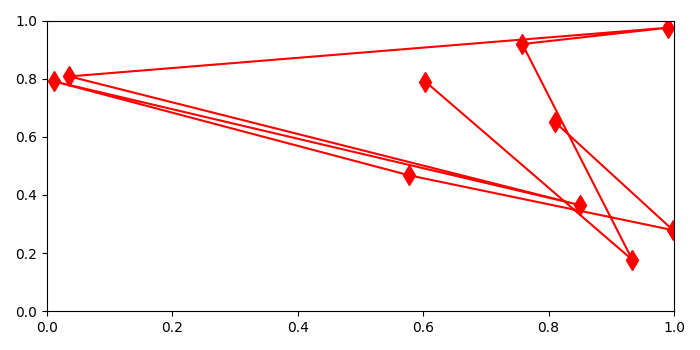# How to retrieve XY data from a Matplotlib figure?

To retrieve XY data from a matplotlib figure, we can use get_xdata() and get_ydata() methods.

## Steps

• Create x and y data points using numpy.

• Limit X and Y axes range, using xlim() and ylim() methods.

• Plot xs and ys data points using plot() method with marker=diamond, color=red, and markersize=10, store the returned tuple in a line.

• Use get_xdata() and get_ydata() methods on the line to get xy data.

• To display the figure, use show() method.

## Example

import numpy as np
from matplotlib import pyplot as plt
plt.rcParams["figure.figsize"] = [7.00, 3.50]
plt.rcParams["figure.autolayout"] = True
xs = np.random.rand(10)
ys = np.random.rand(10)
plt.xlim(0, 1)
plt.ylim(0, 1)
line, = plt.plot(xs, ys, marker='d', c='red', markersize=10)
xdata = line.get_xdata()
ydata = line.get_ydata()
print("X-data of plot is: {}Y-data for plot is: {}".format(xdata, ydata))
plt.show()

## Output

When we execute the code, it will display a plot and print its XY data on the console.

X-data of plot is: [0.80956382 0.99844606 0.57811592 0.01201992 0.85059459
0.03628843 0.99122502 0.7581602 0.93371784 0.60358098]
Y-data for plot is: [0.65190208 0.27895754 0.46742327 0.79049074 0.36485545
0.80771822 0.9753513 0.91897778 0.17651205 0.7898951 ]